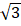# From a point within an equilateral triangles perpendiculars drawn to the three sides are 6 cm, 7 cm and 8 cm. the length of the side of the triangle is   (a) 7 cm (b) 10.5 cm (c) 14cm (d)cm

## Question ID - 50111 :- From a point within an equilateral triangles perpendiculars drawn to the three sides are 6 cm, 7 cm and 8 cm. the length of the side of the triangle is   (a) 7 cm (b) 10.5 cm (c) 14cm (d)cm

3537Let each side of the triangle becm.

Then, area+ ar () + ar () = ar ().cm.

Next Question :

Ge and Si diodes start conducting at 0.3 V and 0.7 respectively. In the following figure if Ge diode connection are reversed, the value of V changes by: (assume that the Ge diode has large breakdown voltage)a. 0.6 V b. 0.8 V c. 0.4 V d. 0.2 V